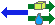## elements & isotopes ▼

How to use:
1. Type a chemical formula
2. Composition, Mass Spec, and elemental analysis will be shown as you type
Reaction calculations (optional):
Alter the type of units as needed before converting
• Converting mass to mmol
• Enter a mass, and click the yellow box arrow to calculate the (m)mol
• Converting volume to mmol (no mass needed)
• Enter a volume, and a density. Click the arrow with the blue droplet to calculate (m)mol
• Converting mmol to volume/mass
• Fill in the density field (if needed), and enter the value of mol/mmol.

# Chemical composition calculationsshare

calculation in progress ...

### Reaction amounts (COSSH forms)

Mass used
unit
volume
unit
density
updateequiv
mmols
unit

C:
H:
N:

### Composition

 Condensed formula Composed of Mass Monoisotopic mass MSDS
Element
Number of Atoms
Atomic mass
Total Mass (amu)
% composition

### [M+] prediction

Predicted Mass Spectrum for the molecular ion [M+].